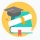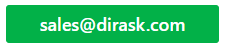Home
IT Knowledge
IT Career
Inspiration
Languages
EN

# Java - generate unique random numbers

3 points
Created by:AnnLen
9180

There is couple of ways to generate unique random numbers in java.

## 1. List with Collections.shuffle

``````List<Integer> list = new ArrayList<>();
for (int i = 1; i <= 10; i++) {
}
Collections.shuffle(list);

// [8, 9, 7, 2, 10, 5, 3, 6, 1, 4]
System.out.println(list);``````
`Collections.shuffle(list)` - java docs:
Randomly permutes the specified list using a default source of randomness.
All permutations occur with approximately equal likelihood.

## 2. HashSet with random numbers between 1 and 20

``````Set<Integer> unique = new HashSet<>();

while (unique.size() != 10) {
}

// [16, 1, 17, 18, 6, 9, 10, 13, 14, 15]
System.out.println(unique.toString());``````

We generate new unique numbers until `HashSet` has expected size.

## 3. Java 8 - Random + IntStream + distinct + limit

``````List<Integer> list = ThreadLocalRandom.current()
.ints(1, 20)
.boxed()
.distinct()
.limit(10)
.collect(Collectors.toList());

// [3, 17, 9, 15, 4, 19, 18, 16, 14, 8]
System.out.println(list);``````

`ints(1, 20)` - generate random numbers between 1 and 20
`limit(10)` - size of our output collection will be 10

## Java - random numbers